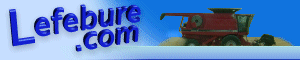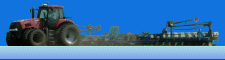Lefebure.com / Software / Delaunay Triangulation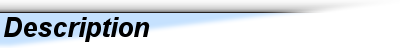Delaunay Triangulation is an algorithm that will take a set of points (X,Y) and build a triangle mesh. The algorithm in this program only works in 2D, but the Z axis can be stored for each vertex for use in displaying a 3D surface. The algorithm is widely published on the Internet, although most examples are in another language such as C++. I ported it to Visual Basic.Net and then added an interface to make mouse clicks add points to the data set and recalculate the mesh.
http://en.wikipedia.org/wiki/Delaunay_triangulation
Note: The processing requirement goes up exponentially as the number of points increases. For less than 500 points, it will compute in less than one second on current hardware. At 10,000 data points, the time to compute will be noticeable (10+ seconds). Also, be sure to do duplicate detection on the data set. Two identical data points will cause odd results.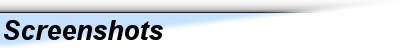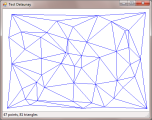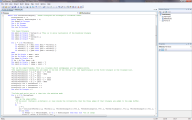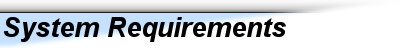- Microsoft Windows 2000 or newer
- Microsoft .Net framework v2.0 or higher
- Microsoft Visual Basic Express 2008 or higher (to view source code)Free, Open SourceCurrent Version: 2009.07.20
- Compiled Program
- Source Code (VB2008)